# Concept/Design Paper on Hybrid Car – Kanhaiya Kumar

Title: Concept/Design Paper on Hybrid Car

Author: Kanhaiya Kumar, 3rd Year, ECE Department

College: SDM College of Engineering & Technology, Dharwad

1. ABSTRACT

As so far we are using conventional energy sources, as a fuel in internal combustion engine in our vehicles. After few years our non-renewable energy sources get exhausted so we must have an alternate energy source to overcome this problem. Now we wish to use electric machine instead of internal combustion engine with the help of transformer and motor.

2. INTRODUCTION
Transformer has two coils primary and secondary there is no electrical connection exists between primary and secondary coils. If primary coil is connected to current source there exists a current in secondary coil also causes linking of flux between primary and secondary coils with magnitude depends on no. of turns of coil and voltage.

3.EFFICIENCY OF INTERNAL COMBUSTION ENGINE
In general fuel consumption and cost of petrol engine is

• Power=25-40 hp.
• Mileage= 15kmpl
• If we drive the car at a speed 100 km per hr. and the mileage of the car is 15kmpl then

In one hour, fuel consumption =7 L ((100÷15) = 6.7 approx.)
Total cost= 75×7= Rs 525.00

For diesel engine car,

• If we use 40 hp engine
• Mileage=10kmpl
• If the speed of car is 100 km/h and the mileage is of 10kmpl

Then consumption of fuel = (100/10)=10 lt/hr.
Total cost = (60×10) =Rs.600.00

• Much fuel consumption.
• Excessive heating of engine if engine works for long time.
• Control on vehicle decreases due decrease in friction at high speed.

4. IDEA
We shall replace engine of a car by the motor and make the car movable with the help of electromagnetic induction principle with the use of transformer. There will be a primary coil lying below the road surface which will get energized with the help of power station and in the same way there will be a secondary coil which is connected at the base of car and just five inches above the secondary coil. We have faradays electromagnetic principal according to which whenever there is relative motion between conductor and a magnetic field, the flux linkage with a coil changes and this change in flux induces a voltage across a coil. If we connect this current carrying wire to motor which is already connected to propeller shaft then we can make the idea in this paper is discussed here.

• We can increase or decrease friction between tyre and road by magnetic field as in maglev vehicles
• Soundless
• Less maintenance required.
• One time investment in coil below the roads
• As IC engine is not present, the cost of the car is less
• Speed of car can be enhanced depending upon roads (more than 250kmph)
• Awesome driving experience.
• Life of engine will increase.
• Environment friendly as no exhaust gases will be produced.

4.2 CONCEPT
The emf generated in secondary coil due to flux change can be given by Lenz law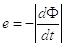Where e is voltage generated;
The voltage generated in coil can be controlled by no of turns of primary and secondary coil which is given by the equation
V1.N2=V2.N1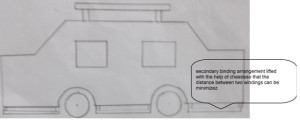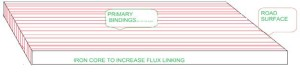4.4 RELATED EQUATIONS AND CALCULATIONV1.N2=V2.N1
e2=-N2 A|dB/dt| ; this is total voltage generated in secondary coil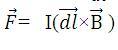where, I = current in wire ; we can make use of this force to control the centrifugal force and friction between tyres and road.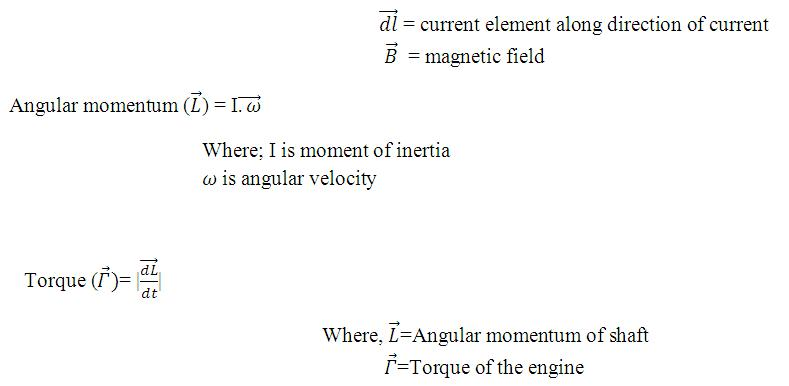To increase the friction between road and tyres and control the centrifugal force
By the Biot-savart’s Law ,
We have ;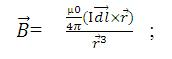Suppose X and Y are two long parallel conductors with currents I1 and I2 respectively. The magnitude of the magnetic field B, at any point onY due to current I1 is given by F=I2 B1L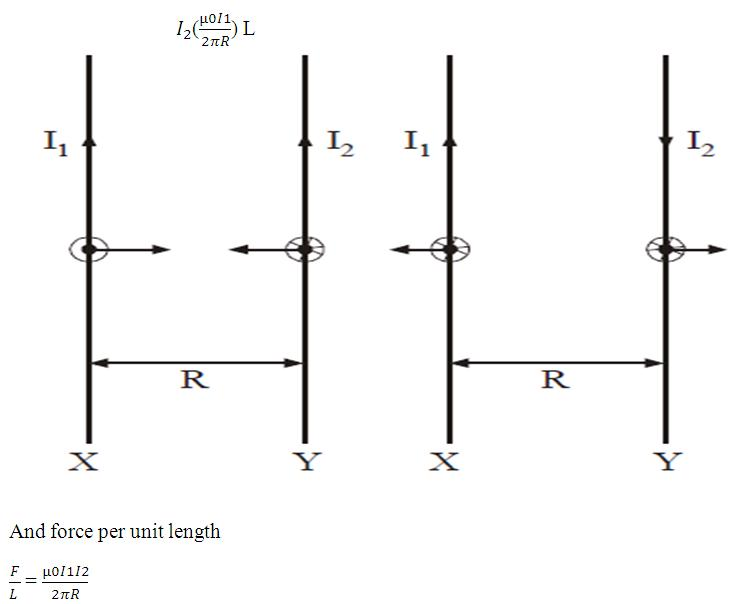According to Flemings left hand rule the direction of this force is towards X when I1 and I2 are in same direction and is away from X when I1 and I2 are in opposite directionSo increasing the current we can increase or decrease magnetic field and hence friction force between tyres and road.

• There are some assumption of magnetic field lines;
• They forms close loops.
• Tangent at any point gives the magnitude and direction at that line
• So, in our simple 220v-12v transformer as the voltage is less. The strength of magnetic line i.e. Of elliptical shape is less.
• Instead of 220 v if we supply higher voltage like 11000v or more in primary coil, then we can increase the strength of magnetic flux lines and can manipulate magnetic flux line to cross secondary coil also
• The efficiency of transformer is now a days more than 95%.so, if we get efficiency more than 50%,that time also it is economical and providing us an alternate source of energy.

Application of magnetic field
To control the speed car.
Let the speed of car is 200km/hr and the diameter of car wheel is 0.5mtr.
The total distance travelled by car in 1hour is 200km=200,000 mtrs.=(200,000÷3600)=55.55mtr/sec.
The perimeter of car’s wheel i= πd= π×0.5mtr=1.57mtr.
Then the total revolution of the wheel in 1second =
( 55.55÷1.57)=35revolution.
At such a high speed tyre surface treated as smooth. This leads to chance of skidding when break is applied.

Velocity of the car is 200mtr/sec. Let the radius of the road is 1km=1000mtr. Then centrifugal force = (mv^2)/r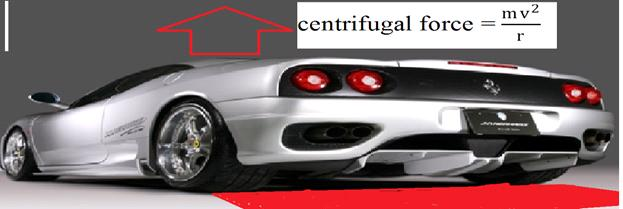Let the weight of car is 1500kg and velocity is 55.55mtr/sec.
Centrifugal force (Fg)=(1500×55.55×55.55)÷1000= 4.628kN on the car directed upward.
Due to such a high force car is raised upward and makes the shape of tyre u-shaped. As break is applied due to less contact area with the road, excessive heat is generated which leads to damage of the tyre and many undesirable things.
If we control centrifugal force by magnetic field, we can drive easily . Let Fb is the magnetic force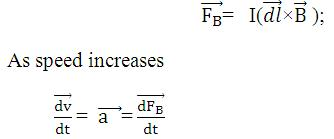If increase the magnetic force with same rate we can make the contact of car’s tyre and the road as much as we want we can control skidding of car.

5. CONCLUSION
This system is a very simple to implement. The calculations above also points out that this idea can be realized practically. In this paper very basic designs had been shown. The advantage of this idea, simple to construct, will make it, even, much useful. The most effective part of this idea is that it is purely eco-friendly. We can also conclude that this idea will help to make systems more efficient and cost effective. If proper attention is made over this idea then we can even implement it practically.

6. REFERENCES
http://en.wikipedia.org/wiki/Lenz_law
http://physicscatalyst.com/magnetism/mag_current_2.php
Concept of physics volume 2 by H.C Verma